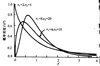ANOVA

Analysis of Variance

• 方差分析

2021-01-20 12:31:02
知识点：方差分析 本周是统计学学习小组-第二期的第十四周，我们这周的学习内容是【方差分析】，涉及到的二级知识点有两个，分别是： 1、基本概念：因子、水平 2、方差分析分类：单因素方差分析、双因素方差分析、...
•方差分析
• ******** 警告 ********* 该程序最初是在 MATLAB 不支持重复测量方差分析时发布的。 但是，自几个版本之前，MATLAB 统计工具箱已添加此功能（请参阅 fitrm 函数）。 因此，该程序现已弃用，不再推荐。 问题是它只...matlab
• 求解多因素一元方差分析，适用于spass中的方差分析多因素方差分析
• 详解单因素方差分析、多因素方差分析、正交实验设计及代码实现.pdf
• 利用半方差分析方法，进行区域变量的空间自相关性分析，了解其空间变异特征
• 数学建模国赛获奖论文整理，使用方差分析做的论文集合，可以系统的学习方差分析在数学建模中的应用，非常有用。
• 在 MATLAB 中执行重复测量或混合方差分析可能非常乏味。 一旦您掌握了 Wilkinson 表示法和 MATLAB fitrm 和 Ranova 要求的相当不寻常的程序，您仍然需要每次创建多个表。 总是有可能使用 MATLAB Central 上可用的...matlab
• 三因素多元方差分析，胡想顺，刘小凤，目的 给出三因素多元方差分析的原理以及过程。方法 在学习两因素多元方差分析的基础上进行三因素多元方差分析的过程推导。结果 �
• 方差分析是用于两个及两个以上样本均数差别的显著性检验。方差分析中，由于各种因素的影响，研究所得的数据呈现波动状，这种波动可以分为组间波动和组内波动两种情况。 食物1 食物2 食物3 3 5 5 2 3 6 1 ...
• 方差分析(Analysis of Variance，简称ANOVA)，又称“变异数分析”或“F检验”，用于两个及两个以上样本均数差别的显著性检验。双因素方差分析即影响试验的因素有两个，且分为无交互作用和有交互作用两种情况。
• 利用半方差分析方法，进行区域变量的空间自相关性分析，了解其空间变异特征
• 统计学第十四周 ：方差分析 一、知识点 因子 在方差分析中所要检验的对象称为因子或因素factor。如书中所介绍消协分析不同行业的服务质量，因此行业就可以称为因子。不同行业如零售业、旅游业、航空公司、家电制造业...
• 主要包括中因素方差分析即完全随机设计或成组设计的方差分析，和两因素方差分析即配伍组设计的方差分析。 　方差分析位于函数选板的“数学→概率与统计→假没捡验”，如图所示。 　如图　方差分析子选板 　如表...
• ####双因素方差分析，可进行有交互作用与无交互作用 def f_twoway(df_c,col_fac1,col_fac2,col_sta,interaction=False): df=df_c.copy() list_fac1=df[col_fac1].unique() list_fac2=df[col_fac2].unique() r=...
• R语言计算平衡不完全区组设计(BIB)的方差分析与多重对比；有助于农林业研究进行BIB试验的方差分析与多重对比！！！包含数据处理格式，没有使用R语言中有关BIB的packages，自己编码；代码简单易懂，执行无错误！ ...
•r语言
• 多因素方差分析的自由度

千次阅读 2021-04-22 06:35:27
下面是做anova的matlab命令，注意matlab软件自带的命令只提供完全随机设计方差分析，要做重复测量方差分析需要到mathworks官网下载 1. 完全随机设计单因素方差分析 anova1 2. 完全随机设计两因素方差分析 anova2 3....

在脑科学中，通常采用重复测量的方式来开展研究。也就是设计不同的任务，让同一个被试都做一遍，来找出差异。这样做不仅是为了节约经费(想想如果4个条件，每个条件找20人，就得给出80人的被试费！)，另一方面也是尽量将个体差异进行控制，因为不管是EEG/ERP、还是fMRI，都是噪声富聚的信号，而且个体差异也是非常大的。图1 要点在于被试间和被试内进行分解

当然，重复测量也有其弊端，如滞留效应，潜隐效应和学习效应，这里不展开来说。重复测量方差分析可以说是我们最常用到的工具。比如：要研究不同情绪图片，在回忆和再认任务中在各个电极上theta波的差异。那就是一个情绪(高兴、悲伤和中性)×任务(回忆、再认)×电极(4个电极)实验，即3因素方差分析。如果把被试分为两组，分别完成回忆和再认任务，这就更复杂了，成了混合设计的3因素方差分析。比较可悲的是，翻阅很多教科书，都重点讲单因素方差分析，或者是完全随机设计的方差分析。对我们常用的重复测量多因素方差分析，保持了集体的沉默。看了许多研究报告，发现同学们在写F(x,y)=???,

P=???，通常把自由度x,y写错。这里我总结一下常用的实验设计如何确定自由度，希望对大家实践有帮助。

(注意本讲义不包括因素无交互作用的情况，亦不包括自由度要矫正的情况！)

分解要诀：方差分析多因素

先看设计定思路

重复与否是关键

项目数定总自由度

先把被试来数数

定出被试间自由度

混合设计要小心

组间因素分解出

被试内有自由度

总减被试间可得出

定下被试内因素

误差自然不会误

核心思想：把总自由度分解为被试间(between

subject)自由度和被试内(within subject)自由度

1

重复测量单因素方差分析被试数:n 组内因素：a

总自由度：an-1

被试间自由度:n-1

被试内自由度：n(a-1)

*因素：a-1

*因素×被试间：(n-1)(a-1)

生成的结果：

F(a-1,(n-1)(a-1))

2

重复测量2因素方差分析被试数:n 组内因素：a,b

总自由度：abn-1

被试间自由度：n-1

被试内自由度：n(ab-1)

*因素a：a-1

*因素b：b-1

*交互：(a-1)(b-1)

*因素a×被试间：(n-1)(a-1)

*因素b×被试间：(n-1)(a-1)

*因素a×因素b×被试间：(n-1)(a-1)

生成的结果：

F(a-1,(n-1)(a-1))

F(b-1,(n-1)(b-1))

F((a-1)(b-1),(n-1)(a-1)(b-1))

3

混合设计(既有组间，又有组内的)2因素方差分析被试数:n 组间因素：a 组内因素：b

总：abn-1

被试间:an-1

*组间因素:a-1

*被试:a(n-1)

被试内：an(b-1)

*组内因素：b-1

*组内×组间：(a-1)(b-1)

*误差：a(b-1)(n-1)

生成的结果：

F(a-1,a(n-1))

F(b-1,a(b-1)(n-1))

4

重复测量3因素方差分析，这在脑科学研究中非常常用！

被试数:n 组内因素：a,b,c

总：abcn-1

被试间：n-1

被试内：n(abc-1)

*因素a：a-1

*因素b：b-1

*因素c：c-1

*交互ab：(a-1)(b-1)

*交互bc：(b-1)(c-1)

*交互ac：(a-1)(c-1)

*交互abc：(a-1)(b-1)(c-1)

*因素a×被试间：(a-1)(n-1)

*因素ab×被试间：(a-1)(b-1)(n-1)

*因素abc×被试间：(a-1)(b-1)(c-1)(n-1)

生成的结果：

F(a-1,(a-1)(n-1))

F(b-1,(b-1)(n-1))

F((a-1)(b-1),(a-1)(b-1)(n-1))

F((a-1)(b-1)(c-1),(a-1)(b-1)(c-1)(n-1))

5

混合设计(既有组间，又有组内的)3因素方差分析如果a因素处理的学习效应很强，无法采用方案4，通常采用方案5来避免学习效应，因此，本方案在脑科学研究中也很常用！

被试数:n 组间因素：a 组内因素：b,c

总：abcn-1

被试间：an-1

*组间因素a: a-1

*被试：a(n-1)

被试内自由度：an(bc-1)

*组内因素b：b-1

*组内因素c：c-1

*交互ab：(a-1)(b-1)

*交互bc：(b-1)(c-1)

*交互ac：(a-1)(c-1)

*交互abc：(a-1)(b-1)(c-1)

*误差：a(bc-1)(n-1)

生成的结果：

F(a-1,a(n-1))

F(b-1,a(bc-1)(n-1))

6

注意和完全随机设计的区别，举例如下：

被试数:abn 组间因素：a,b

如果把abn个被试随机分到a,b两个因素下,就构成了完全随机设计的2因素方差分析。

分解顺序和重复测量也不同，没有被试间和被试内这一步，变成了直接进行处理间和处理内分解。

总自由度：abn-1

处理间:ab-1

*因素a：a-1

*因素b：b-1

*交互ab：(a-1)(b-1)

*处理内：ab(n-1)

生成的结果：

F(a-1,ab(n-1))

F(b-1,ab(n-1))

7

如果把abcn个被试随机分到a,b,c三个因素下,就构成了完全随机设计的3因素方差分析。

直接进行处理间和处理内分解。

总：abcn-1

处理间:abc-1

*因素a：a-1

*因素b：b-1

*因素c：c-1

*交互ab：(a-1)(b-1)

*交互bc：(b-1)(c-1)

*交互ac：(a-1)(c-1)

*交互abc：(a-1)(b-1)(c-1)

*处理内：abc(n-1)

生成的结果：

F(a-1,abc(n-1))

F(b-1,abc(n-1))

给出了以上自由度，我们来证明一个观点：“同样的实验因素和每个处理下的被试量，完全随机比混合设计自由度高，混合设计比重复测量自由度高”。取3因素方差分析为例：

完全随机：a和b

F(a-1,abc(n-1))

F(b-1,abc(n-1))

混合设计：组间a和组内b

F(a-1,a(n-1))

F(b-1,a(bc-1)(n-1))

重复测量：a和b

F(a-1,(a-1)(n-1))

F(b-1,(b-1)(n-1))

可以看到，对于a因素：abc(n-1)>a(n-1)>(a-1)(n-1)；

对于b因素：abc(n-1)>a(bc-1)(n-1)>(b-1)(n-1)；

所以上面的观点是成立的。还可以看到，对于混合设计：a(n-1)<=a(bc-1)(n-1)，也就是在a=b时，组内条件比组间条件自由度大。

练习题：

为了验证自己有没有掌握，建议完成以下练习题：

1.混合设计3因素方差分析被试数:n

组间因素：a,b

组内因素：c

2.混合设计3因素方差分析考虑每组被试数不等的情况，a=2为被试间因素，被试数在每种处理下具体为：n1

(a1条件) n2 (a2条件)

组间因素：a

组内因素：b, c

3.重复测量5因素方差分析

被试数：n

组内因素：a,b,c,d,e

你知道答案吗？

下面是做anova的matlab命令，注意matlab软件自带的命令只提供完全随机设计方差分析，要做重复测量方差分析需要到mathworks官网下载

1. 完全随机设计单因素方差分析

anova1

2. 完全随机设计两因素方差分析

anova2

3. 完全随机设计多因素方差分析

anovan

4. 重复测量单因素方差分析

5. 重复测量两因素方差分析

6. 重复测量三因素方差分析

7. 混合设计两因素方差分析(既有组间，又有组内)

点击命令可直接下载

下面是实验数据统计分析的常规路线图展开全文•方差分析
• R语言方差分析，是一个成功分析的代码，大家想学习交流的可以看看R语言
• 双因素和多因素方差分析，Stata软件教学视频，适合多学科学习和教学stata
• Allan方差分析的m代码文件，里面有可以测试的data.mat文件，主要步骤在allan.m和nihe.m文件中
• 两因素等重复试验下的方差分析在药物剂量分析中的应用，谢瑜，王传美，为寻求一种治疗枯草热病的药物中两种成分剂量的最佳组合，进行两因素等重复试验。首先对试验数据进行相关分析和偏相关分析，结果...